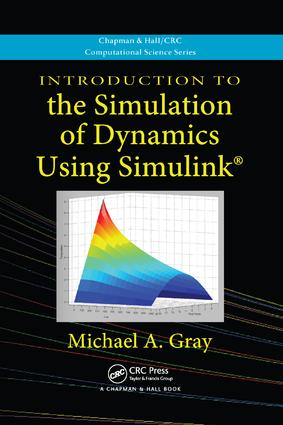# Introduction to the Simulation of Dynamics Using Simulink

## 1st Edition

Chapman and Hall/CRC

332 pages | 32 Color Illus. | 211 B/W Illus.

##### Purchasing Options:\$ = USD
Paperback: 9781138114708
pub: 2017-06-07
\$82.95
x
Hardback: 9781439818978
pub: 2010-07-02
\$220.00
x
eBook (VitalSource) : 9780429184307
pub: 2010-07-02
from \$39.98

FREE Standard Shipping!

### Description

Designed for undergraduate students in the general science, engineering, and mathematics community, Introduction to the Simulation of Dynamics Using Simulink® shows how to use the powerful tool of Simulink to investigate and form intuitions about the behavior of dynamical systems. Requiring no prior programming experience, it clearly explains how to transition from physical models described by mathematical equations directly to executable Simulink simulations.

Teaches students how to model and explore the dynamics of systems

Step by step, the author presents the basics of building a simulation in Simulink. He begins with finite difference equations and simple discrete models, such as annual population models, to introduce the concept of state. The text then covers ordinary differential equations, numerical integration algorithms, and time-step simulation. The final chapter offers overviews of some advanced topics, including the simulation of chaotic dynamics and partial differential equations.

A one-semester undergraduate course on simulation

Written in an informal, accessible style, this guide includes many diagrams and graphics as well as exercises embedded within the text. It also draws on numerous examples from the science, engineering, and technology fields. The book deepens students’ understanding of simulated systems and prepares them for advanced and specialized studies in simulation. Ancillary materials are available at http://nw08.american.edu/~gray

Introduction and Motivation

Systems

Dynamical Models of Physical Systems

Constructing Simulations from Dynamical Models

How Simulators Are Used

The Basics of Simulation in Simulink

Simplest Model to Simulate

Simulation of the Simplest Model

Understanding How Time Is Handled in Simulation

A Model with Time as a Variable

How Simulink Propagates Values in Block Diagrams

A Model with Uniform Circular Motion

A Model with Spiraling Circular Motion

Uncertainty in Numbers and Significant Figures

Simulation of First-Order Difference Equation Models

What Is a Difference Equation?

Examples of Systems with Difference Equation Models

First-Order Difference Equation Simulation

Examining the Internals of a Simulation

Organizing the Internal Structure of a Simulation

Using Vector and Matrix Data

Simulation of First-Order Differential Equation Models

What Is a Differential Equation?

Examples of Systems with Differential Equation Models

Reworking First-Order Differential Equations into Block Form

First-Order Differential Equation Simulation

Saving Simulation Data in MATLAB

Fixed-Step Solvers and Numerical Integration Methods

What Is a Solver?

Understanding the Basics of Numerical Integration Algorithms

Understanding Solver Errors

Improving the Basic Algorithms

Fixed-Step Solvers in the Simulink Software

Simulation of First-Order Equation Systems

What Is a First-Order Difference Equation System?

Examples of First-Order Difference Equation Systems

Simulating a First-Order Difference Equation System

What Is a First-Order Differential Equation System?

Examples of First-Order Differential Equation Systems

Simulating a First-Order Differential Equation System

Combining Connections on a Bus

Simulation of Second-Order Equation Models: Nonperiodic Dynamics

Simulation of Second-Order Difference Equation Models

Simulation of Second-Order Differential Equation Models

Second-Order Differential Equation Models with First-Order Terms

Conditional Dynamics

Simulation of Second-Order Equation Models: Periodic Dynamics

Orbital Systems

Creating Libraries

Higher-Order Models and Variable-Step Solvers

Direct Simulation by Multiple Integrations

Producing Function Forms for Simulation Results

Variable-Step Solvers

Variable-Step Solvers in Simulink

Advanced Topics: Transforming Ordinary Differential Equations, Simulation of Chaotic Dynamics, and Simulation of Partial Differential Equations

Transforming Ordinary Differential Equations

Simulation of Chaotic Dynamics

Simulation of Partial Differential Equations

Appendix A: Alphabetical List of Simulink Blocks

Appendix B: The Basics of MATLAB for Simulink Users

Appendix C: Debugging a Simulink Model

Index

A Summary, References, and Additional Reading appear at the end of each chapter.

### About the Author

Michael A. Gray is an associate professor in the Department of Computer Science at American University in Washington, D.C.

### Subject Categories

##### BISAC Subject Codes/Headings:
MAT000000
MATHEMATICS / General
MAT021000
MATHEMATICS / Number Systems
TEC007000
TECHNOLOGY & ENGINEERING / Electrical